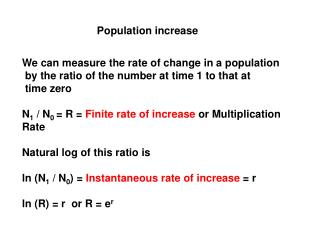DownloadDownload PresentationPopulation increase

# Population increase

Télécharger la présentation## Population increase

- - - - - - - - - - - - - - - - - - - - - - - - - - - E N D - - - - - - - - - - - - - - - - - - - - - - - - - - -
##### Presentation Transcript

1. Population increase We can measure the rate of change in a population by the ratio of the number at time 1 to that at time zero N1 / N0 = R = Finite rate of increase or Multiplication Rate Natural log of this ratio is ln (N1 / N0) = Instantaneous rate of increase = r ln (R) = r or R = er

2. Population increase For t time intervals r = ln (N t / N0)/ t or r = ln (Rt)/ t

3. Population increase • Therefore, Nt / N 0 = Rt = e rt • Nt = N0 . e rt • ln (Nt) = ln N0 + r t • This is a straight line with slope of r • Underconditions of • Superabundant food, ii) optimal climate, iii) no predators, then • r is maximum = r m or r max • r m = Intrinsic rate of increase. It is a feature of the species

4. Population increase Taking the Intrinsic rate of increase, then Nt = N0 . e rm t Differentiate this and we get dN/ dt = rm N = Population rate of increase dN / dt. 1/N = rm = Per capita rate of increase All of these versions show an exponential rate of increase

5. Population increase So far we have assumed optimum conditions. This is unrealistic. We need a term that causes the population to level off, mimicking competition. dN/ dt = rm N (1 – N / K) This is called the LOGISTIC EQUATION where the term (1 – N/K) is an index of how far N is from its maximum K and modifies rm so that when N = K dN/dt = 0 K is the carrying capacity or maximum population size.

6. Population increase the LOGISTIC EQUATION dN/ dt = rm N (1 – N / K) Produces an S-shaped curve where K is the asymptote dN/dt. 1/N = rm (1-N/K) = r r is the Instantaneous rate of increase again and can be any value from rm to zero

7. Instantaneous rate of change in Yellowstone Elk

8. Population increase • Does the LOGISTIC EQUATION fit observations? • This is simply a mathematical mimic. Do we see populations behaving this way? • Laboratory experiments. • Gause 1934 with Paramecium studies

9. Gause’s 1934 experiments

10. Population increase Does the LOGISTIC EQUATION fit observations? 2. Field observations Brent geese in France Sheep in Tasmania

11. Population increase Does the LOGISTIC EQUATION fit observations? Assumes immediate responses to conditions. It does not incorporate time lags. 3. Time lags occur Reindeer on Pribilov islands Snowshoe hares and lynx in northern Canada

12. REINDEER ON PRIBILOV ISLANDS OFF ALASKA

13. Population increase Does the LOGISTIC EQUATION fit observations? Assumes immediate responses to conditions. It does not incorporate time lags. 4. Seasonal changes – some pops respond to seasonal changes in climate, especially insects. Rapid increase in summer and decrease in winter Thrips on roses in Australia Daphnia in Katherine Lake BC